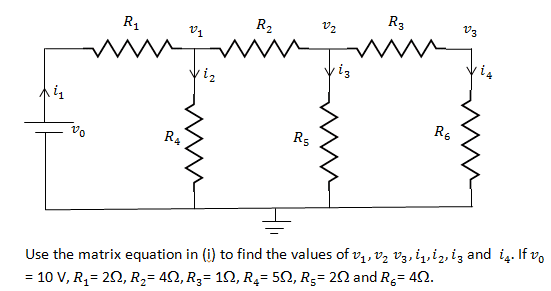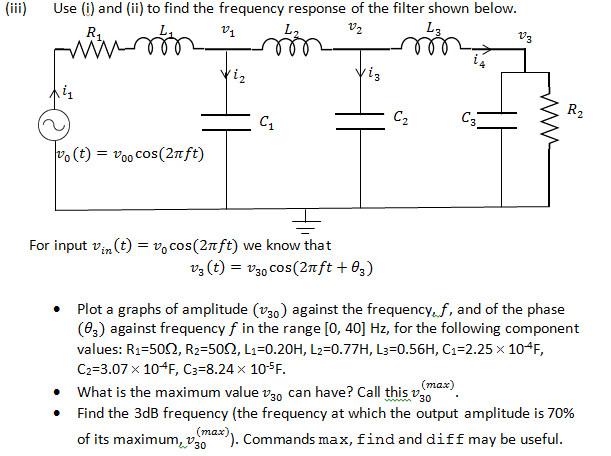# Calculating the frequency response of filter with a matrix

## Homework Statement

This is actually part 3 of the question. Part one was to form 7 equations to form a 7x7 matrix, part 2 was to solve it, which I've done. This question is to be done with Matlab, by the way.Part 3:## Homework Equations

Frequency response = Vout / Vin.

## The Attempt at a Solution

I know the frequency response is the ratio of Vout and Vin, in this case, v3 and v0, I'm not sure how to incorporate that with the matrix I have. I could replace the resistor values with the impedance of the corresponding elements, but since the frequency is unknown, that wouldn't work. I've calculated frequency responses before, but that was for simpler circuit in which I could use the potential divider formula.

donpacino
Gold Member
calculating the frequency response at one frequency would get you one one point of the graph.
What if you compute 10 different frequencies?
What if you compute 100?
or 1000?

you can still use the same equations you used for the previous parts.

By the way you might want to show everyone the equations you got